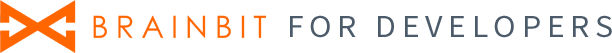# Filters

## Description

It is a cross-platform library that allows you to filter the signal with a given set of filters for a specified sampling rate.

The library provides built-in filters of the following types:
1. high pass - high pass filter (HPF). Cuts off all frequencies below the filter pass frequency
2. low pass - low pass filter (LPF). Cuts off all frequencies above filter pass frequency.
3. band pass - bandpass filter. Passes a certain bandwidth
4. band stop is a band reject filter. Suppresses a certain band.

Digital signal processing involves the formation of the necessary bandwidth and suppression of network interference. The bandwidth is formed by sequential application of VFD and LPF filters. The HPF removes the constant component of the signal and the drift of the isoline, attracting the signal to 0. The LPF removes high-frequency noise from the signal. Mains interference caused by mains interference usually has the frequency of the mains itself - 50 Hz for Russia and CIS countries and 60 Hz for America and some other countries. Mains interference often has a very high amplitude signal and even when outside the signal bandwidth it still gives significant distortion, which leads to the need to suppress it with regenerative filters.

Maximum available signal bandwidth directly depends on sampling frequency and according to Nyquest law does not exceed half of sampling frequency. In practice, usually guided by the rule - Fh=Fd/4. Thus, if we have a sampling frequency of 1000 Hz, the maximum signal frequency will be 250 Hz. This value can be pushed higher, up to 400 Hz, but the higher the frequency, the lower the accuracy of the signal transmission will be.

Thus, a typical signal processing chain is the use of LPF, LPF and the required number of notch filters. The order in which the filters are applied does not matter.

Each filter has a set of internal coefficients, which are recalculated after each new sample of the signal. This means that you cannot use the same instance of the filter class to process multiple signals at once. The rule is 1 filter - 1 signal works here.

## Types

### FilterType

Type of filter, enum with values:
1. FtHP - high pass filter
2. FtLP - low pass filter
3. FtBandStop - band stop filter
4. FtBandPass - band pass filter
5. FtNone - default value, type not set

### FilterParam

Parameters of filter, structure with fields:
1. type - type of filter
2. samplingFreq - sampling frequency of raw signal, integer value
3. cutoffFreq - central frequency, double value

## Preinstalled filters

Library contains a list of some filters. The full list you can get with functions:

``````int fcount = 0;
get_preinstalled_filter_count(&fcount);

FilterParam* preinstallFilters = new FilterParam[fcount];
get_preinstalled_filter_list(preinstallFilters);```
```
````FilterParam[] preinstallFilters = PreinstalledFilters.List();`
```
````preinstalled_filters = preinstalled_filters_list()`
```
```
```
```
```
```
```
```
```
```
```

## Initialization

For work with filter you need to create a `Filter` object and optionally `FilterList` object. The `FilterList` contains objects of the `Filter` type and manipulates them.

``````// creating filter
FilterParam param;
param.type = FtBandStop;
param.samplingFreq = 250;
param.cutoffFreq = 50.0f;
TFilter* filter = create_TFilter_by_param(param);

// then create list of filters
TFilterList* flist = create_TFilterList();

```
``````// creating filter
FilterParam param;
param.type = FtBandStop;
param.samplingFreq = 250;
param.cutoffFreq = 50.0;
Filter filter = new Filter(param);

// then create list of filters
FilterList flist = new FilterList();

```
``````# creating filter
filter = Filter()
param = FilterParam(FilterType.ft_band_stop, 250, 100)
filter.init_by_param(param)

# then create list of filters
filter_list = FilterList()

```
```
```
```
```
```
```
```
```
```
```

## Work with library

### Filter one value:

``````// by one filter
double sample = SAMPLE;
double filteredValue = TFilter_Filter(filter, sample);

// by list of filters
double sample = SAMPLE;
double filteredValue = TFilterList_Filter(flist, sample);```
```
``````// by one filter
double sample = SAMPLE;
double filteredValue = filter.Filter(sample);

// by list of filters
double sample = SAMPLE;
double filteredValue = flist.Filter(sample);```
```
``````sample = SAMPLE
filteredValue = flist.Filter(sample)```
```
```
```
```
```
```
```
```
```
```
```

### Filter array of values:

``````// by one filter
double samples[max_samples];
TFilterList_Filter_array(filter, samples, max_samples);

// by list of filters
double samples[max_samples];
TFilterList_Filter_array(flist, samples, max_samples);```
```
``````// by one filter
double[] samples = new double[max_samples];
filter.FilterArray(samples);

// by list of filters
double[] samples = new double[max_samples];
flist.FilterArray(samples);```
```
``````# by one filter
samples = [x for x in range(max_samples)]
filter.filter_array(samples);

# by list of filters
samples = [x for x in range(max_samples)]
flist.filter_array(samples);```
```
```
```
```
```
```
```
```
```
```
```

## Finishing work with the library

``````// removing filters
delete_TFilter(filter);

// removing list of filters
delete_TFilterList(flist);```
```
``````// removing filters
filter.Dispose();

// removing list of filters
flist.Dispose();```
```
``````# removing filters
del filter

# removing list of filters
del flist```
```
```
```
```
```
```
```
```
```
```
```